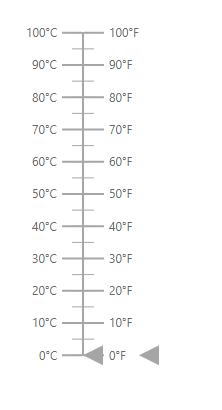Search results

# Axes in ASP.NET MVC Linear Gauge control

25 Oct 2021 / 6 minutes to read

Axis is used to indicate the numeric values in the linear scale. The Linear Gauge component can have any number of axes. The sub-elements of an axis are line, ticks, labels, ranges, and pointers.

## Setting the start value and end value of the axis

The start value and end value for the Linear Gauge can be set using the `Minimum` and `Maximum` properties in the `LinearGaugeAxis` respectively. By default, the start value of the axis is 0 and the end value of the axis is 100.

razor
``````@using Syncfusion.EJ2.LinearGauge

@Html.EJS().LinearGauge("gauge").Axes(new List<Syncfusion.EJ2.LinearGauge.LinearGaugeAxis>
{
new Syncfusion.EJ2.LinearGauge.LinearGaugeAxis
{
Minimum=20, Maximum=200
}
}).Render()``````

<<<<<<< HEAD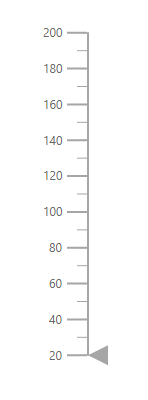=======25c536e4a77a22da0ab7e1d0d5fc4cc724190ae6

## Line Customization

The following properties in the `LinearGaugeLine` can be used to customize the axis line in the Linear Gauge.

razor
``````@using Syncfusion.EJ2.LinearGauge;

@Html.EJS().LinearGauge("gauge").Axes(new List<Syncfusion.EJ2.LinearGauge.LinearGaugeAxis>
{
new Syncfusion.EJ2.LinearGauge.LinearGaugeAxis
{
Line = new Syncfusion.EJ2.LinearGauge.LinearGaugeLine
{
Height = 150, Width= 2, Color = "#4286f4", Offset = 2
}
}
}).Render()``````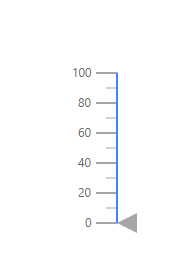## Ticks Customization

Ticks are used to specify the interval in the axis. Ticks are of two types, major ticks and minor ticks. The following properties in the `LinearGaugeMajorTicks` and `LinearGaugeMinorTicks` can be used to customize the major ticks and minor ticks respectively.

razor
``````@using Syncfusion.EJ2;

@Html.EJS().LinearGauge("gauge").Axes(new List<Syncfusion.EJ2.LinearGauge.LinearGaugeAxis>
{
new Syncfusion.EJ2.LinearGauge.LinearGaugeAxis
{
MajorTicks = new Syncfusion.EJ2.LinearGauge.LinearGaugeTick
{
Height=10, Width=2, Interval=20, Color="red"
},
MinorTicks = new Syncfusion.EJ2.LinearGauge.LinearGaugeTick
{
Height = 5, Width = 2, Interval = 5, Color = "Orange"
}
}
}).Render()``````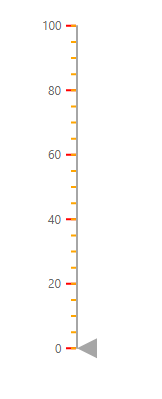### Positioning the ticks

The minor and major ticks can be positioned by using the `Offset` and `Position` properties. The `Offset` is used to render the ticks with the specified distance from the axis. By default, the offset value is 0. The possible values of the `Position` property are Inside, Outside, Cross, and Auto. By default, the ticks will be placed inside the axis.

razor
``````@using Syncfusion.EJ2.LinearGauge

@Html.EJS().LinearGauge("gauge").Axes(new List<Syncfusion.EJ2.LinearGauge.LinearGaugeAxis>
{
new Syncfusion.EJ2.LinearGauge.LinearGaugeAxis
{
MajorTicks = new Syncfusion.EJ2.LinearGauge.LinearGaugeTick
{
Height=10, Width=2, Interval=20, Color="red", Position = Position.Outside
},
MinorTicks = new Syncfusion.EJ2.LinearGauge.LinearGaugeTick
{
Height = 5, Width = 2, Interval = 5, Color = "Orange", Position = Position.Cross
}
}
}).Render()``````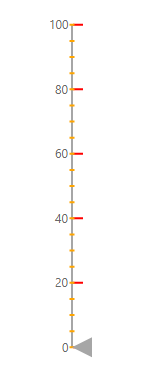## Labels Customization

The style of the labels can be customized using the following properties in `LinearGaugeFont` in the `LinearGaugeLabel`.

razor
``````@using Syncfusion.EJ2.LinearGauge;

@Html.EJS().LinearGauge("gauge").Axes(new List<Syncfusion.EJ2.LinearGauge.LinearGaugeAxis>
{
new Syncfusion.EJ2.LinearGauge.LinearGaugeAxis
{
LabelStyle = new Syncfusion.EJ2.LinearGauge.LinearGaugeLabel
{
Font = new LinearGaugeFont
{
FontFamily = "Roboto",
Size = "12px",
Color = "red",
Opacity = 1,
FontWeight = "Regular"
}
}
}
}).Render()``````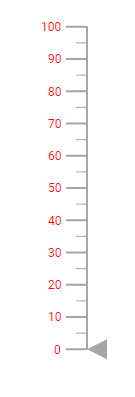### Positioning the axis label

Labels can be positioned by using `Offset` and `Position` properties in the `LinearGaugeLabel`. The `Offset` defines the distance between the labels and ticks. By default, the offset value is 0. The possible values of `Position` property are Inside, Outside, Cross, and Auto. By default, the labels will be placed inside the axis.

razor
``````@using Syncfusion.EJ2.LinearGauge

@Html.EJS().LinearGauge("gauge").Axes(new List<Syncfusion.EJ2.LinearGauge.LinearGaugeAxis>
{
new Syncfusion.EJ2.LinearGauge.LinearGaugeAxis
{
LabelStyle = new Syncfusion.EJ2.LinearGauge.LinearGaugeLabel
{
Position = Position.Cross
}
}
}).Render()``````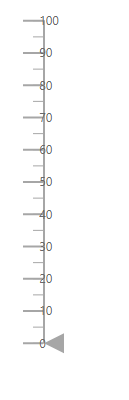### Customizing the display of the last label

If the last label is not in the visible range, it will be hidden by default. The last label can be made visible by setting the `ShowLastLabel` property as true in the `LinearGaugeAxis`.

razor
``````@using Syncfusion.EJ2;

@Html.EJS().LinearGauge("gauge").Axes(new List<Syncfusion.EJ2.LinearGauge.LinearGaugeAxis>
{
new Syncfusion.EJ2.LinearGauge.LinearGaugeAxis
{
Minimum = 0, Maximum = 115, ShowLastLabel = true
}
}).Render()``````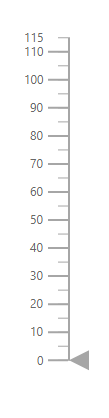### Label Format

Axis labels in the Linear Gauge control can be formatted using the `Format` property in `LinearGaugeLabel`. It is used to render the axis labels in a certain format or to add a user-defined unit in the label. It works with the help of placeholder like {value}°C, where value represents the axis value. For example, 20°C.

razor
``````@using Syncfusion.EJ2;

@Html.EJS().LinearGauge("gauge").Axes(new List<Syncfusion.EJ2.LinearGauge.LinearGaugeAxis>
{
new Syncfusion.EJ2.LinearGauge.LinearGaugeAxis
{
LabelStyle = new Syncfusion.EJ2.LinearGauge.LinearGaugeLabel
{
Format = "{value}°C"
}
}
}).Render()``````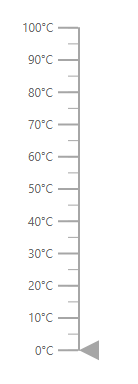### Displaying numeric format in labels

The numeric formats such as currency, percentage, and so on can be displayed in the labels of the Linear Gauge using the `Format` property in the Linear Gauge component. The following table describes the result of applying some commonly used label formats on numeric values.

 Label Value Label Format property value Result Description 1000 n1 1000.0 The number is rounded to 1 decimal place. 1000 n2 1000.00 The number is rounded to 2 decimal place. 1000 n3 1000.000 The number is rounded to 3 decimal place. 0.01 p1 1.0% The number is converted to percentage with 1 decimal place. 0.01 p2 1.00% The number is converted to percentage with 2 decimal place. 0.01 p3 1.000% The number is converted to percentage with 3 decimal place. 1000 c1 * ,000.0 The currency symbol is appended to number and number is rounded to 1 decimal place. 1000 c2 * ,000.00 The currency symbol is appended to number and number is rounded to 2 decimal place.
razor
``````@using Syncfusion.EJ2.LinearGauge;

@Html.EJS().LinearGauge("gauge").Format("c").Axes(new List<Syncfusion.EJ2.LinearGauge.LinearGaugeAxis>
{
new Syncfusion.EJ2.LinearGauge.LinearGaugeAxis
{
}
}).Render()``````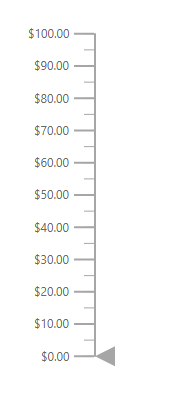## Orientation

By default, the Linear Gauge is rendered vertically. To change its orientation, the `Orientation` property must be set to Horizontal.

razor
``````@using Syncfusion.EJ2.LinearGauge;

@Html.EJS().LinearGauge("gauge").Orientation(Orientation.Horizontal).Axes(new List<Syncfusion.EJ2.LinearGauge.LinearGaugeAxis>
{
new Syncfusion.EJ2.LinearGauge.LinearGaugeAxis
{
Minimum = 20, Maximum = 140
}
}).Render()``````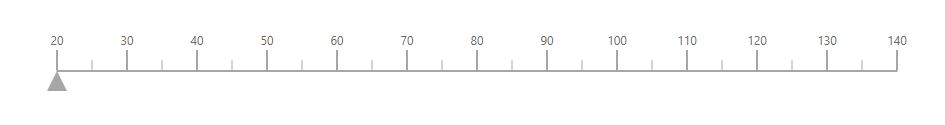## Inverted Axis

The axis of the Linear Gauge component can be inversed by setting the `IsInversed` property to true in the `LinearGaugeAxis`.

razor
``````@using Syncfusion.EJ2.LinearGauge;

@Html.EJS().LinearGauge("gauge").Axes(new List<Syncfusion.EJ2.LinearGauge.LinearGaugeAxis>
{
new Syncfusion.EJ2.LinearGauge.LinearGaugeAxis
{
IsInversed = true
}
}).Render()``````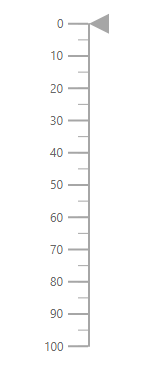## Opposed Axis

To place an axis opposite from its original position, `OpposedPosition` property in the `LinearGaugeAxis` must be set as true.

razor
``````@using Syncfusion.EJ2.LinearGauge;

@Html.EJS().LinearGauge("gauge").Axes(new List<Syncfusion.EJ2.LinearGauge.LinearGaugeAxis>
{
new Syncfusion.EJ2.LinearGauge.LinearGaugeAxis
{
OpposedPosition = true
}
}).Render()``````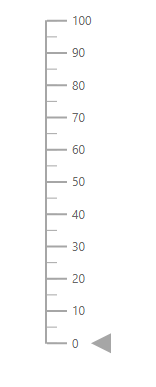## Multiple Axes

Multiple axes can be added to the Linear Gauge by adding multiple `LinearGaugeAxis` in the `LinearGaugeAxes` and customization can be done with the `LinearGaugeAxis`. Each axis can be customized separately as shown in the following example.

razor
``````@using Syncfusion.EJ2.LinearGauge;

@Html.EJS().LinearGauge("gauge").Axes(new List<Syncfusion.EJ2.LinearGauge.LinearGaugeAxis>
{
new Syncfusion.EJ2.LinearGauge.LinearGaugeAxis
{
LabelStyle = new Syncfusion.EJ2.LinearGauge.LinearGaugeLabel
{
Format="{value}°C"
}
},
new Syncfusion.EJ2.LinearGauge.LinearGaugeAxis
{
OpposedPosition = true,
LabelStyle = new Syncfusion.EJ2.LinearGauge.LinearGaugeLabel
{
Format="{value}°F"
}
}
}).Render()``````